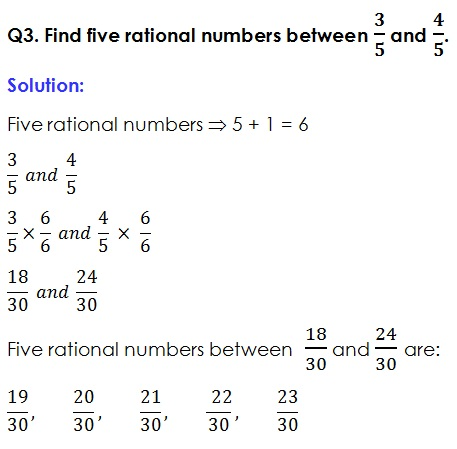Study Materials

# NCERT Solutions for Class 9th Mathematics

Page 1 of 5

## Chapter 1. Number Systems

### Exercise 1.1

Exercise:1.1

Q1. Is zero a rational number? Can you write it in the form p/q, where p and q are integers and q ≠ 0?

Solution:

Yes, zero is a rational number, because it can be represented on a number line and it can be written in the form of p/q,

Where P = 0 and q ≠ 0;Q4. State whether the following statements are true or false. Give reasons for your answers.

(I)   Every natural number is a whole number.

(ii)  Every integer is a whole number.

(iii) Every rational number is a whole number.

Solution:

(I)   Every natural number is a whole number.  (True

Reason: Whole numbers contains all natural numbers.

(ii)  Every integer is a whole number. (False

Reason: Integers have also negative number while whole number does not have such negative numbers.

(iii) Every rational number is a whole number. (False

Reason: Rational numbers have variety of numbers, those numbers can not be represented as whole number.

Page 1 of 5

Chapter Contents: